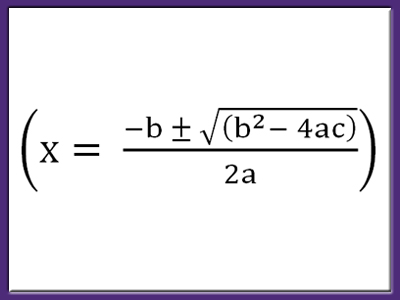This Math quiz is called 'Quadratic Equations' and it has been written by teachers to help you if you are studying the subject at high school. Playing educational quizzes is a user-friendly way to learn if you are in the 9th or 10th grade - aged 14 to 16.

It costs only \$12.50 per month to play this quiz and over 3,500 others that help you with your school work. You can subscribe on the page at Join Us

Quadratic expressions are recognizable as they have an x2 term along with (usually) an x-term and a number. Quadratic equations don’t behave like linear ones – sometimes they don’t even have a solution, yet at other times they can have 2! Quadratic curves, called parabolas, occur in nature and in real-life situations, so it’s a good idea to know all the intricacies of them. See how well you do in this high school Math quiz!

Quadratics are the first in a series of functions called POLYNOMIALS. They all have decreasing powers of x, where the power is always a whole number. The graphs of polynomials are all curves. The curve of a quadratic can be described as U-shaped (or ∩-shaped for negative x2), which means its gradient is always changing. Compare this to the straight line graph, which has a fixed gradient.

You should be able to carry out a few different techniques with quadratic expressions. Factorizing a quadratic is the first step to solving quadratic equations, but remember not all quadratics will factorize! The ‘perfect square’ and the ‘difference of two squares’ are two special cases, and you should learn how to recognize them.

The roots of a quadratic curve, which is where it crosses the x-axis, correspond to the solutions of the quadratic equation.

Completing the square will allow you to identify the coordinates of the turning point. You can complete the square on any quadratic expression, even if it doesn’t cross the x-axis.

For quadratics that look a bit tricky, use the quadratic formula. The discriminant will give you an indication of the nature of any roots, which will tell you if a solution exists or not.

Question 1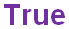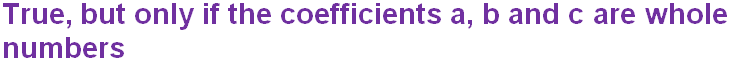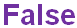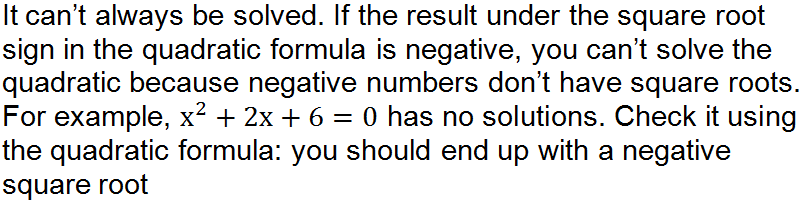Question 2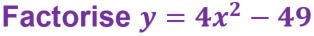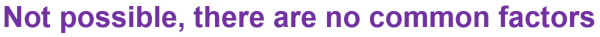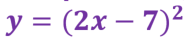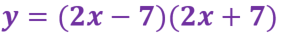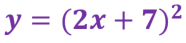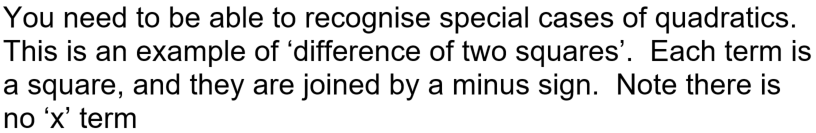Question 3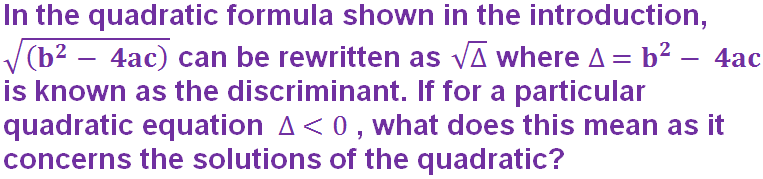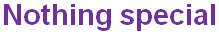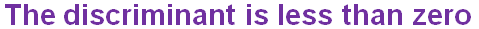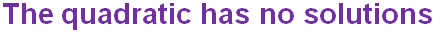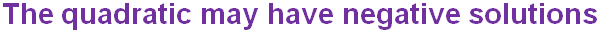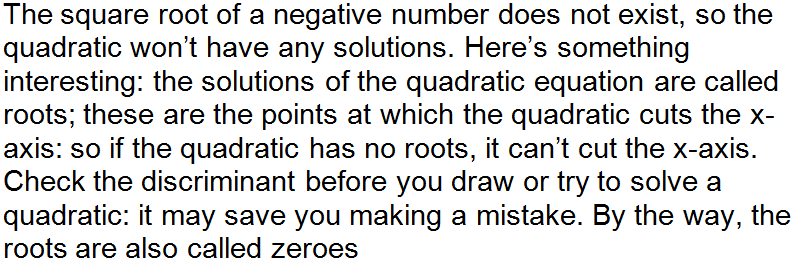Question 4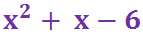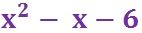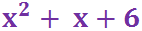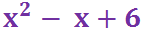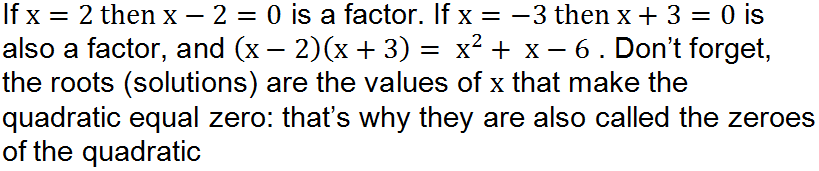Question 5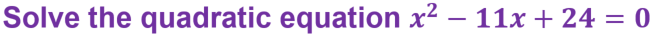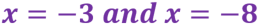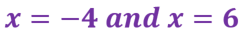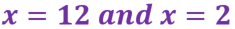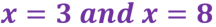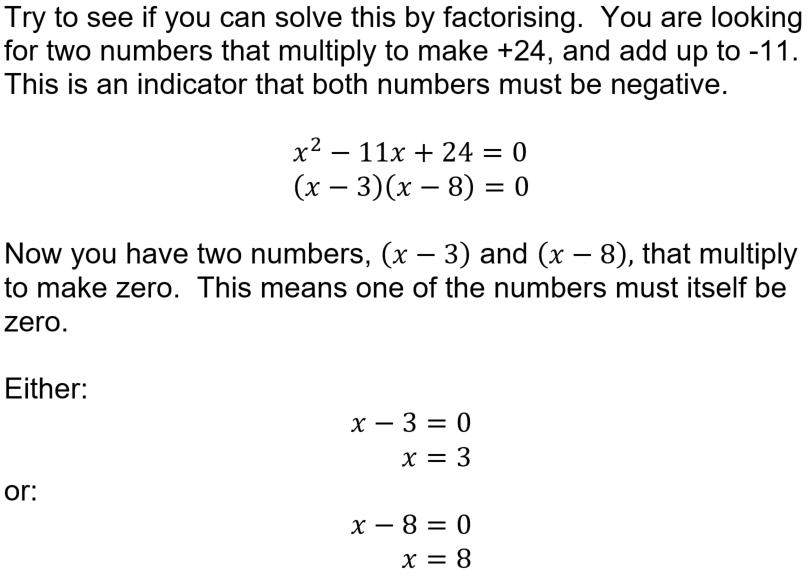Question 6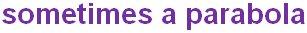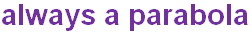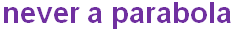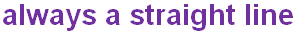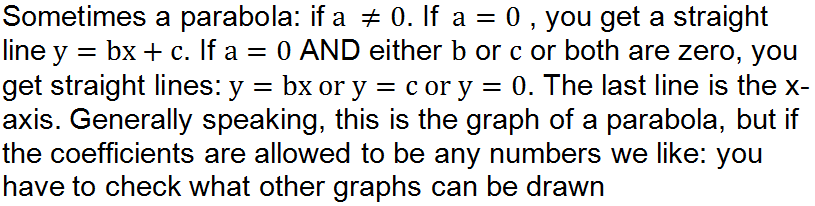Question 7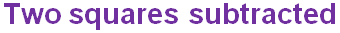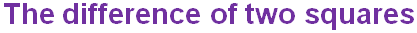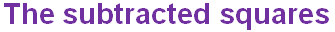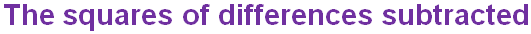Question 8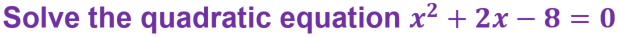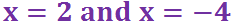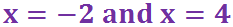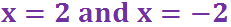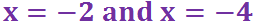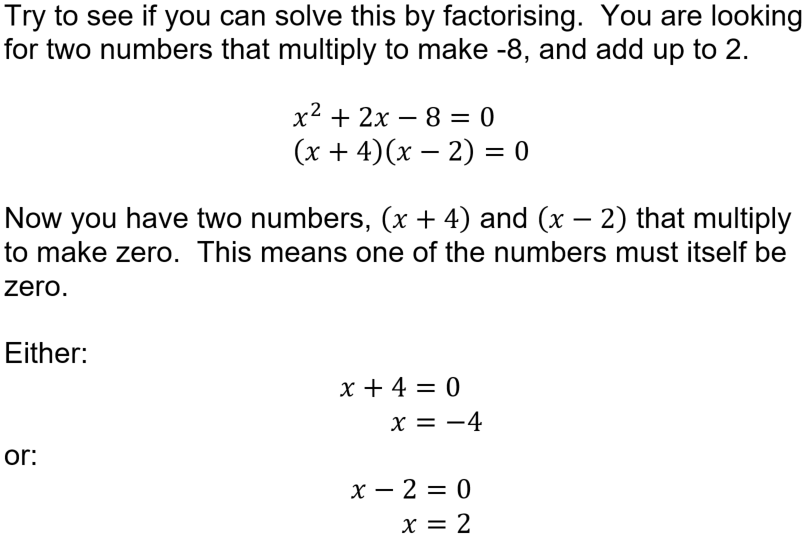Question 9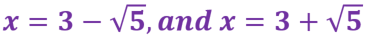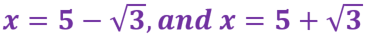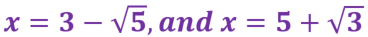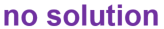Question 10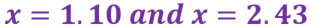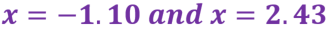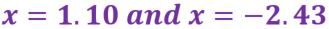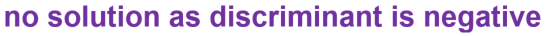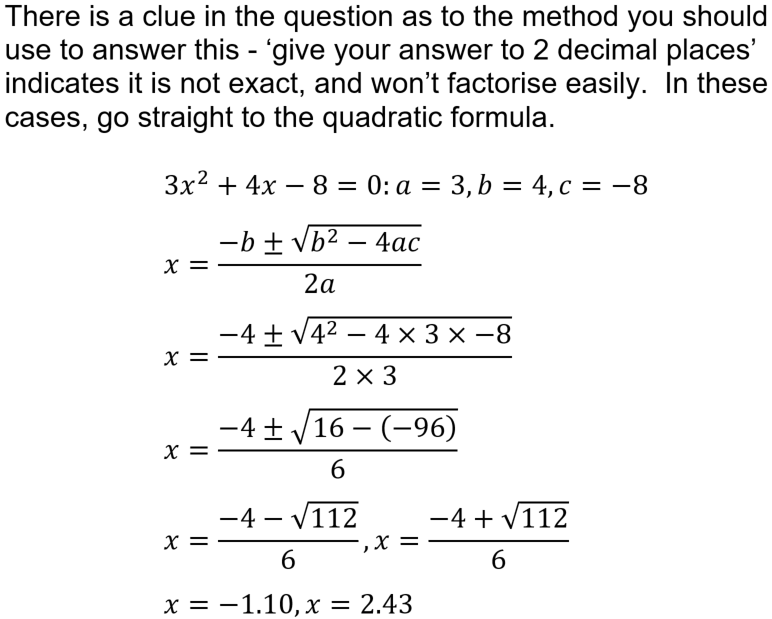Author:  Sally Thompson